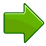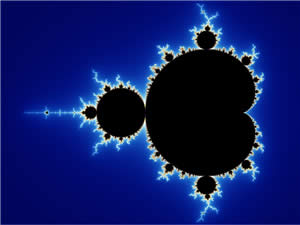# Complex NumbersA Complex Number

A Complex Number is a combination of a
Real Number and an Imaginary NumberReal Numbers are numbers like:

 1 12.38 −0.8625 3/4 √2 1998

Nearly any number you can think of is a Real Number!Imaginary Numbers when squared give a negative result.

Normally this doesn't happen, because:

But just imagine such numbers exist, because we will need them.

The "unit" imaginary number (like 1 for Real Numbers) is i, which is the square root of −1Because when we square i we get −1

i2 = −1

Examples of Imaginary Numbers:

 3i 1.04i −2.8i 3i/4 (√2)i 1998i

And we keep that little "i" there to remind us we need to multiply by √−1

## Complex Numbers

A Complex Number is a combination of a Real Number and an Imaginary Number:### Examples:

 1 + i 39 + 3i 0.8 − 2.2i −2 + πi √2 + i/2

### Can a Number be a Combination of Two Numbers?Can we make up a number from two other numbers? Sure we can!

We do it with fractions all the time. The fraction 3/8 is a number made up of a 3 and an 8. We know it means "3 of 8 equal parts".

Well, a Complex Number is just two numbers added together (a Real and an Imaginary Number).

## Either Part Can Be Zero

So, a Complex Number has a real part and an imaginary part.

But either part can be 0, so all Real Numbers and Imaginary Numbers are also Complex Numbers.

Complex Number Real Part Imaginary Part
3 + 2i 3 2
5 5 0 Purely Real
−6i 0 −6 Purely Imaginary

## Complicated?Complex does not mean complicated.

It means the two types of numbers, real and imaginary, together form a complex, just like a building complex (buildings joined together).

## A Visual Explanation

You know how the number line goes left-right?

Well let's have the imaginary numbers go up-down:And we get the Complex Plane

A complex number can now be shown as a point:The complex number 3 + 4i

## Adding

To add two complex numbers we add each part separately:

(a+bi) + (c+di) = (a+c) + (b+d)i

### Example: add the complex numbers 3 + 2i and 1 + 7i

• add the real numbers, and
• add the imaginary numbers:

(3 + 2i) + (1 + 7i)
= 3 + 1 + (2 + 7)i
= 4 + 9i

Let's try another:

### Example: add the complex numbers 3 + 5i and 4 − 3i

(3 + 5i) + (4 − 3i)
= 3 + 4 + (5 − 3)i
= 7 + 2i

On the complex plane it is:## Multiplying

To multiply complex numbers:

Each part of the first complex number gets multiplied by
each part of the second complex number

Just use "FOIL", which stands for "Firsts, Outers, Inners, Lasts" (see Binomial Multiplication for more details):Firsts: a × c Outers: a × di Inners: bi × c Lasts: bi × di (a+bi)(c+di) = ac + adi + bci + bdi2

Like this:

### Example: (3 + 2i)(1 + 7i)

(3 + 2i)(1 + 7i) = 3×1 + 3×7i + 2i×1+ 2i×7i
= 3 + 21i + 2i + 14i2
= 3 + 21i + 2i − 14   (because i2 = −1)
= −11 + 23i

And this:

### Example: (1 + i)2

(1 + i)(1 + i)= 1×1 + 1×i + 1×i + i2
= 1 + 2i − 1   (because i2 = −1)
= 0 + 2i

### But There is a Quicker Way!

Use this rule:

(a+bi)(c+di) = (ac−bd) + (ad+bc)i

Example: (3 + 2i)(1 + 7i) = (3×1 − 2×7) + (3×7 + 2×1)i = −11 + 23i

### Why Does That Rule Work?

It is just the "FOIL" method after a little work:

(a+bi)(c+di) =ac + adi + bci + bdi2   FOIL method
=ac + adi + bci − bd   (because i2 = −1)
=(ac − bd) + (ad + bc)i   (gathering like terms)

And there we have the (ac − bd) + (ad + bc)i  pattern.

This rule is certainly faster, but if you forget it, just remember the FOIL method.

### Let us try i2

Just for fun, let's use the method to calculate i2

### Example: i2

We can write i with a real and imaginary part as 0 + i

i2 = (0 + i)2= (0 + i)(0 + i)
= (0×0 − 1×1) + (0×1 + 1×0)i
= −1 + 0i
= −1

And that agrees nicely with the definition that i2 = −1

So it all works wonderfully!

Learn more at Complex Number Multiplication.

## Conjugates

We will need to know about conjugates in a minute!

A conjugate is where we change the sign in the middle like this:A conjugate is often written with a bar over it:

### Example:

5 − 3i   =   5 + 3i

## Dividing

The conjugate is used to help complex division.

The trick is to multiply both top and bottom by the conjugate of the bottom.

### Example: Do this Division:

2 + 3i4 − 5i

Multiply top and bottom by the conjugate of 4 − 5i :

2 + 3i4 − 5i×4 + 5i4 + 5i  =  8 + 10i + 12i + 15i216 + 20i − 20i − 25i2

Now remember that i2 = −1, so:

=  8 + 10i + 12i − 1516 + 20i − 20i + 25

Add Like Terms (and notice how on the bottom 20i − 20i cancels out!):

=  −7 + 22i41

Lastly we should put the answer back into a + bi form:

=  −7 41 + 2241i

DONE!

Yes, there is a bit of calculation to do. But it can be done.

## Multiplying By the Conjugate

There is a faster way though.

In the previous example, what happened on the bottom was interesting:

(4 − 5i)(4 + 5i) = 16 + 20i − 20i − 25i2

The middle terms (20i − 20i) cancel out! Also i2 = −1 so we end up with this:

(4 − 5i)(4 + 5i) = 42 + 52

Which is really quite a simple result. The general rule is:

(a + bi)(a − bi) = a2 + b2

We can use that to save us time when do division, like this:

### Example: Let's try this again

2 + 3i4 − 5i

Multiply top and bottom by the conjugate of 4 − 5i :

2 + 3i4 − 5i×4 + 5i4 + 5i  =  8 + 10i + 12i + 15i216 + 25

=  −7 + 22i41

And then back into a + bi form:

=  −7 41 + 2241i

DONE!

## Notation

We often use z for a complex number. And Re() for the real part and Im() for the imaginary part, like this:Which looks like this on the complex plane:## The Mandelbrot SetThe beautiful Mandelbrot Set (pictured here) is based on Complex Numbers. It is a plot of what happens when we take the simple equation z2+c (both complex numbers) and feed the result back into z time and time again. The color shows how fast z2+c grows, and black means it stays within a certain range. Here is an image made by zooming into the Mandelbrot setAnd here is the center of the previous one zoomed in even further:Challenging Questions: 1 2